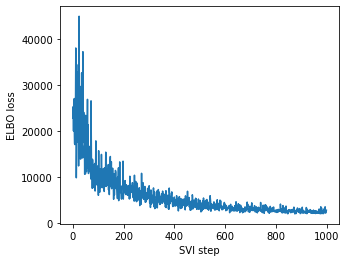# User warning: Singular sample detected

While I’m constructing a model, I get a warning message

``````UserWarning: Singular sample detected.
warnings.warn("Singular sample detected.")
``````

I can run the SVI to perform approximate inference, like this (with 1000 iterations)But with more iterations I will get into trouble, seems like with the covariance matrix:

``````_LinAlgError: torch.linalg_cholesky: (Batch element 15): The factorization could not be completed because the input is not positive-definite (the leading minor of order 2 is not positive-definite).
``````

In my original model, I used samples from a `Wishart` distribution to be the precision_matrix. Is this the cause of my trouble? (I have been suggested to choose `LKJCholesky` for prior in models.)

what line of source code is generating that warning?

generally i’d suggest using 64 bit precision for numerical linear algebra if you’re not already

This happens when I render or perform inference for the mixture model with Wishart distributed covariance parameter.

``````T = 10
def mix_weights(beta):
beta1m_cumprod = (1 - beta).cumprod(-1)

def model_wishart(data):
alpha = pyro.param("alpha", torch.tensor([1.0]))

with pyro.plate("sticks", T-1):
beta = pyro.sample("beta", Beta(1, alpha))
with pyro.plate("component", T):
mu = pyro.sample("mu", MultivariateNormal(torch.zeros(d), 5 * torch.eye(d)))
cov = pyro.sample("cov", Wishart(df=d, covariance_matrix=torch.eye(d)))
with pyro.plate("data", N):
z = pyro.sample("z", Categorical(mix_weights(beta)))
pyro.sample("obs", MultivariateNormal(mu[z], precision_matrix=cov[z]), obs=data)
model = model_wishart
pyro.render_model(model, model_args=(data,), render_params=True)
``````

Running the code above raises:

``````c:\Users\19046\.conda\envs\pytorch\lib\site-packages\torch\distributions\wishart.py:247: UserWarning: Singular sample detected.
warnings.warn("Singular sample detected.")
``````

However, when I change the prior and replace Wishart with LKJ using a decomposition strategy, this warning simply disappears. Is this related with the model?

this is happening in pytorch code i suggest you look at wishart.py for more information about what “singular” samples are

Oh, I’ll check it out. Thanks for your help!